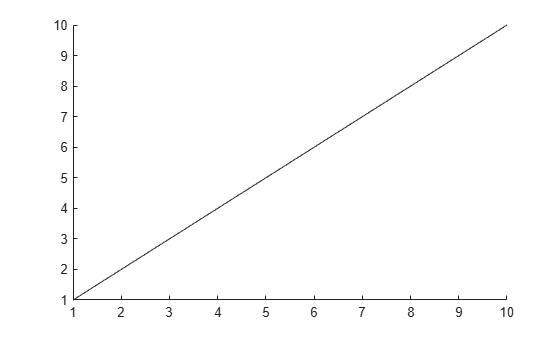# getpoints

Return points that define animated line

## Syntax

``````[x,y] = getpoints(an)``````
``````[x,y,z] = getpoints(an) ``````

## Description

example

``````[x,y] = getpoints(an)``` returns the x and y coordinates for the points that define the animated line specified by `an`. Create an animated line with the `animatedline` function.```
``````[x,y,z] = getpoints(an) ``` returns the coordinates for the 3-D animated line specified by `an`. If the line does not have any z values, then `getpoints` returns `z` as a vector of zeros the same length as `x` and `y`.```

## Examples

collapse all

Create an animated line with 10 points. Then, return the points stored in the animated line.

`h = animatedline(1:10,1:10);``[x,y] = getpoints(h)`
```x = 1×10 1 2 3 4 5 6 7 8 9 10 ```
```y = 1×10 1 2 3 4 5 6 7 8 9 10 ```

## Input Arguments

collapse all

`AnimatedLine` object. Create an `AnimatedLine` object using the `animatedline` function.

## Output Arguments

collapse all

First coordinate values that define the animated line, returned as a vector. For Cartesian axes, the first coordinate is x-axis position. For geographic axes, the first coordinate is latitude in degrees.

Second coordinate values that define the animated line, returned as a vector. For Cartesian axes, the second coordinate is y-axis position. For geographic axes, the second coordinate is longitude in degrees.

Third coordinate values that define the 3-D animated line, returned as a vector. For Cartesian axes, the third coordinate is z-axis position.

## Version History

Introduced in R2014b